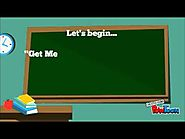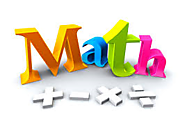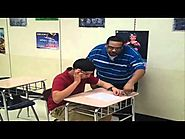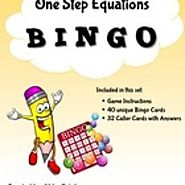Updated by Ahna Werts on May 24, 2016REPORTAhna Werts
Owner
10 items   2 followers   0 votes   30 views

# Solving One-Step Equations!

Here is a list of my favorite resources for learning all about evaluating one-step equations...

9

## Evaluating Expressions: Distance, Rate, and TimeThis video podcast is my own creation, and allows students to illustrate higher order thinking skills by practicing substitution in order to evaluate expressions and one-step equations.

10

## How to Solve One-Step EquationsThis video by the Virtual Math Tutor is a thorough lesson on how to solve one-step equations using inverse operations.

5

## Keeping it in Balance: One Step EquationsA Balancing Act: Using the properties of equality to solve for a variable in a one-step algebraic equation. Students may access this page from Michelle Braggs (which includes an Opening, Work Session, and Closing) through Better Lesson.

7Practice solving equations in one step by adding or subtracting the same value from both sides. This interactive math practice for the concept of solving one-step equations using addition and subtraction provides immediate feedback for users. Very productive and engaging practice opportunity!

2

## Math Rap Dougie - Teach Me How to Solve It (Equations)Students can enjoy learning the concept of one-step equations though Mr. Riley's "Math Rap Dougie". The concept may become easier to students when it is placed to a familiar beat!

8

## One Step Equations: Bingo Game - FREEThis fun activity will surely engage students, and is a great way to practice their skills in solving one step equations through the game of BINGO! Student are required to create a free account to access this free resource.

1

## QuizletThrough the Quizlet website, students have the option to create a free account to use tools and resources, such as interactive flashcards, to become experts at writing and solving one-step equations.

3

## Solving One-Step EquationsThis online-interative Jeopardy game is a self-paced, engaging opportunity for students to illustrate competency in applying inverse operations to solve one-step equations.

4

## Writing Algebraic Equations to Represent Real-World Scenarios (One-Step)Students will translate verbal expressions into algebraic equations to represent real-world problems starting by correctly identifying the variables and operations in the situation. Students may access this lesson from Carla Seeger through Better Lesson.

6

## Writing and Solving EquationsThis is a lesson relating the concept of writing and solving one-step equations to real-world situations. This lesson from Andrea Palmer includes an Opening, Work Session, and Closing.